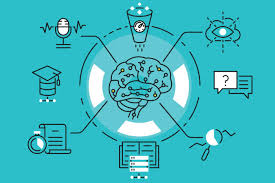Machine Learning

04.05.2020

For the Machine Learning definition go here

The Machine learning ALGORITHMS

Linear Regression

It is used to estimate real values (cost of houses, number of calls, total sales, etc) based on continuous variables.

Logistic Regression

Don't get confused by its name! It is a classification, not a regression algorithm. It is used to estimate discrete values ( Binary values like 0/1, yes/no, true/False) based on a given set of independent variables (s).

In simple words, it predicts the probability of occurrence of an event by fitting data to a logit function. Hence, it is also known as a logit regression. Since it predicts the probability, its output values lie between 0 and 1 (as expected).

Decision Trees

It is a type of supervised learning algorithm that is mostly used for classification problems.

Surprisingly, it works for both categorical and continuous dependent variables. In this algorithm, we split the population into two or more homogeneous sets.

The best way to understand how the decision tree works, is to play Jezzball- a classic game from Microsoft. Essentially, you have a room with moving walls and you need to create walls such that the maximum area gets cleared off without the balls.

Support Vector Machines

A Support Vector Machine (SVM) is a discriminative classifier formally defined by a separating hyperplane.

In other words, given labeled training data (supervised learning), the algorithm outputs an optimal hyperplane that categorizes new examples.

Naive Bayes

It is a classification technique based on Bayes' theorem with an assumption of independence between predictors.

In simple terms, a Naive Bayes classifier assumes that the presence of a particular feature in a class is unrelated to the presence of any other feature. For example, a fruit may be considered to be an apple if it is red, round, and about three inches in diameter.

k Nearest Neighours

It can be used for both classification and regression problems.

However, it is more widely used in classification problems in the industry. k-nearest neighbor is a simple algorithm that stores all available cases and classifies new cases by a majority vote of its k-neighbors.

The case is assigned to the class is most common amongst its k-nearest neighbors measured by a distance function.

K-Means Clustering

It is a type of unsupervised algorithm which solves the clustering problem.

Its procedure follows a simple and easy way to classify a given data set through a certain number of clusters (assume k-clusters). Data points inside a cluster are homogeneous and heterogeneous to peer groups.

Random Forest

Random Forest is a trademarked term for an ensemble of decision trees.

In Random Forest, we have a collection of decision trees (so known as "fores"). To classify a new object based on attributes, each tree gives a classification and we say the tree "votes" for that class.

Dimensionality Reduction

As a data scientist, the data we are offered also consists of many features, this sounds good for building a good robust model but there is a challenge.